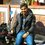# " 2/10 = 2 !! " The rules of Maths may not approve !

Maths has long been developed from "Deductive reasoning and rationality " like all other sciences,though a bit differently . In the midst of a no. of intelligent and mathematically acceptable proofs for a no. of thoughts , today i went on to prove : 2/10 = 2 [not caring much about the general rules....i hope u will like it] So, we 've to prove:
$\frac{2}{10}$ = 2

Starting with L.H.S , TWO/TEN = $\frac{2}{10}$

                       Now assuming   that  'Abc  = A(b + c) ' ,we proceed as follows :

Eliminating the common T , we get,

wo/en


Now , wo/en = (23 +15) / (5 +14) = $\frac{38}{19}$ = $\boxed{2}$

I know this is unacceptable to the rules of maths...... But for the sake of proving any desired thought ...i think , it is certainly nice! i hope u may like it....Note by Tanmay Goyal
6 years, 4 months ago

This discussion board is a place to discuss our Daily Challenges and the math and science related to those challenges. Explanations are more than just a solution — they should explain the steps and thinking strategies that you used to obtain the solution. Comments should further the discussion of math and science.

When posting on Brilliant:

• Use the emojis to react to an explanation, whether you're congratulating a job well done , or just really confused .
• Ask specific questions about the challenge or the steps in somebody's explanation. Well-posed questions can add a lot to the discussion, but posting "I don't understand!" doesn't help anyone.
• Try to contribute something new to the discussion, whether it is an extension, generalization or other idea related to the challenge.
• Stay on topic — we're all here to learn more about math and science, not to hear about your favorite get-rich-quick scheme or current world events.

MarkdownAppears as
*italics* or _italics_ italics
**bold** or __bold__ bold
- bulleted- list
• bulleted
• list
1. numbered2. list
1. numbered
2. list
Note: you must add a full line of space before and after lists for them to show up correctly
paragraph 1paragraph 2

paragraph 1

paragraph 2

[example link](https://brilliant.org)example link
> This is a quote
This is a quote
    # I indented these lines
# 4 spaces, and now they show
# up as a code block.

print "hello world"
# I indented these lines
# 4 spaces, and now they show
# up as a code block.

print "hello world"
MathAppears as
Remember to wrap math in $$ ... $$ or $ ... $ to ensure proper formatting.
2 \times 3 $2 \times 3$
2^{34} $2^{34}$
a_{i-1} $a_{i-1}$
\frac{2}{3} $\frac{2}{3}$
\sqrt{2} $\sqrt{2}$
\sum_{i=1}^3 $\sum_{i=1}^3$
\sin \theta $\sin \theta$
\boxed{123} $\boxed{123}$

## Comments

There are no comments in this discussion.

×

Problem Loading...

Note Loading...

Set Loading...